# Algorithmic worlds

## -

Back to the information page

Introduction
Algorithmic art
Pictorial algorithms
Ultra Fractal
Algorithmic worlds
Piling patterns
The structure
Pattern generators
Index operators
The piling operator
An example
Other modules

## The piling operator

This module is the core of the piling algorithm. The piling operator is an atypical module, in the sense that it uses a whole tree of modules as input (see the figure here). This tree of module by itself produces a pattern. The role of the piling operator is to generate copies at various scales of this pattern, and combine them. (An example was shown here.)

We now discuss some parameters which modify the way the pattern is piled, and therefore alter the appearance of the final image.

The small scale copies of the pattern are usually damped compared to the large scale copies, to avoid the final picture becoming too noisy. The most natural damping is a power law: the amplitude of a pattern is proportional to some power, traditionnaly called beta (B), of its characteristic size. The parameter B has a direct interpretation from an artisitic point of view: it governs the smoothness of the picture. Here are three examples: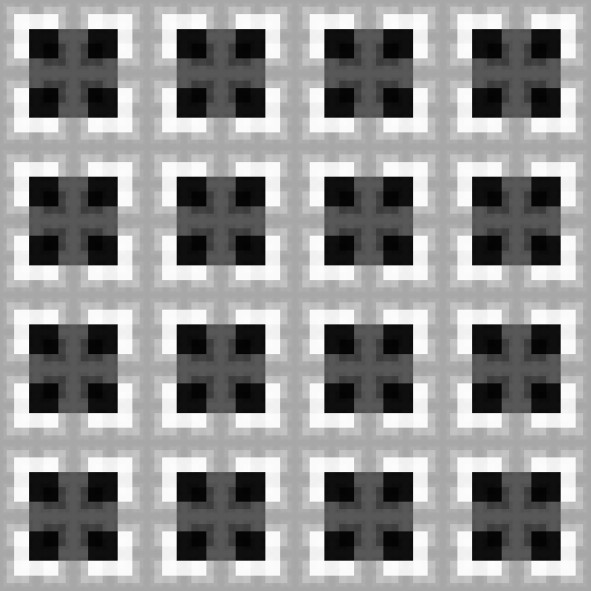This image uses B = 2. A pattern half as large as a given one is damped by a factor of four.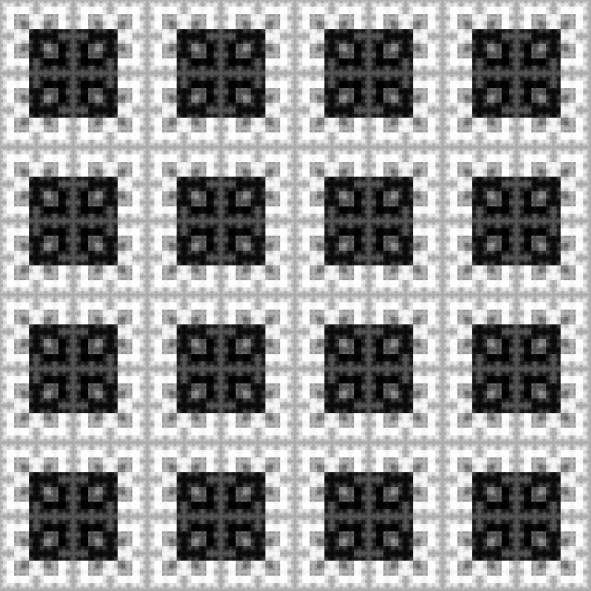This image uses B = 1. The damping is by a factor of two.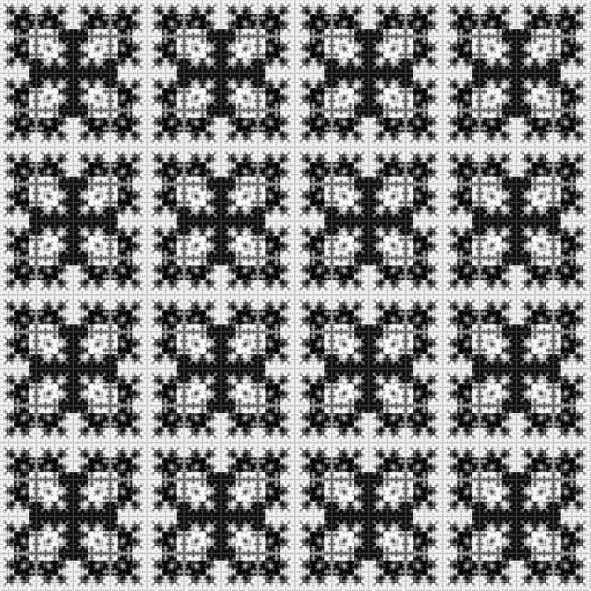Here B = 0.5, so the damping is proportional to the square root of the frequency. Clearly, the higher B is, the smoother the picture looks.

The parameter B is what could be called an artistically relevant parameter. Its value alters the picture in a clear and foreseeable way, so it provides the artist with a useful tool. The discovery and the implementation of artistically relevant parameters are of fundamental importance during the creation of an algorithm. Without such parameters, the algorithm is useless. It is a difficult task, because the parameters having a clear mathematical interpretation are not always artistically relevant. Finding a good set of parameters is one of the artistic aspect of writing algorithms.

There is another interesting tunable parameter, the magnification step. We always mentioned that copies of the pattern "at various scales" should be piled. Practically, each copy of the pattern is obtained from the preceding one by a scaling by a fixed factor, that we call the magnification step. This image uses a magnification step of 2. Indeed, the largest D-shapes are twice as big as the next to largest ones, which are themselves twice as big as the next to next to largest, and so on. This image uses a magnification factor of 4. The largest squares are four times as big as the next to largest ones. The reader can check that the magnification factor is equal to 3 for this image, and to 5 for this image.

Finally, shifting the smaller copies of the pattern can appreciably change the resulting image. Consider the following three images. The first shows a basic pattern. The second and the third show the result of piling it, the two piling differing by a shift of the smaller copies of the pattern.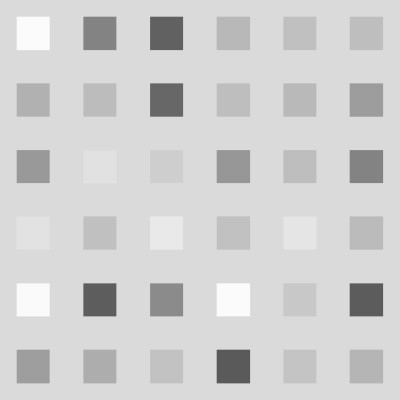The basic pattern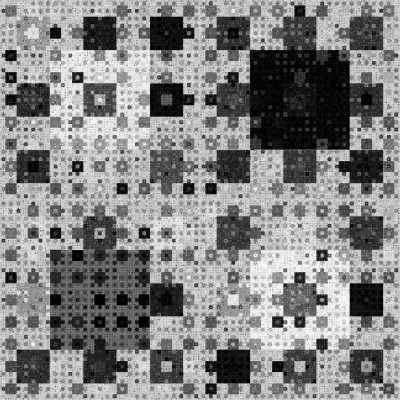The basic pattern has been piled up with a magnification step of 3, without any shift.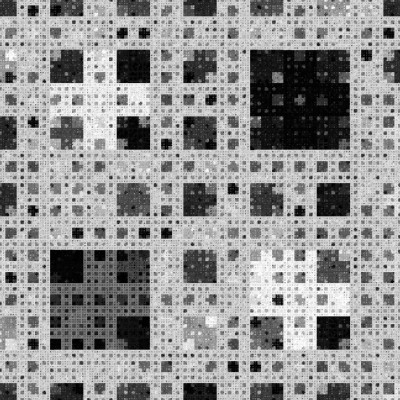The same piling as above, but each copy of the pattern has been shifted horizontally and vertically by the length of the square. The resulting image is noticeably different.

 Copyright S.Monnier 2009-2020. Bookmark or share